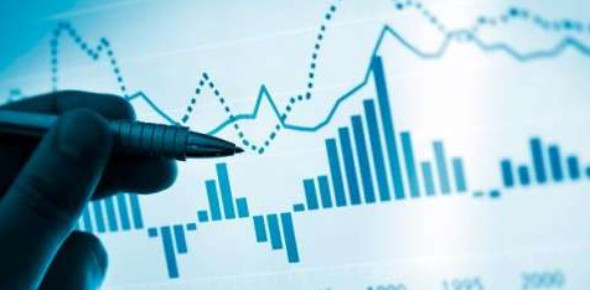# Economics Ultimate Exam: Quiz! MCQ

20 Questions | Total Attempts: 69SettingsAre you eager to take the economics exam? This quiz can initiate excitement for economics. With this quiz, you should appreciate the following topics: how the growth economy is measured, define recession, what has been the average employment rate of the last fifty years, how inflation is measured, what is the federal reserve, and what is macro level. This quiz will show you the type of questions that might be in the exam. All the best.

• 1.
How is growth in the economy measured?
• A.

Consumer price index (CPI)

• B.

Growth Domestic Pressure (GDP)

• C.

Gross Domestic Product (GDP)

• 2.
What does GDP measure?
• A.

The total value of all real estate, imports and consumer goods in a particular country

• B.

The market value of all goods and services produced within a country in a given period.

• C.

Changes in the price level of consumer goods and services purchased by households.

• 3.
Approximately what is the historic average annual rate of GDP over the last 50 years?
• A.

1%

• B.

3%

• C.

5%

• 4.
What is the generally accepted definition of a recession?
• A.

2 consecutive quarters of negative GDP

• B.

3 consecutive quarters of negative GDP

• C.

4 consecutive quarters of negative GDP

• 5.
What are the 2 primary indicators used to measure the level of employment in the United States?
• A.

Unemployment rate and the future hiring estimates

• B.

Unemployment rate and the number of workers hired by the federal government

• C.

Unemployment rate and the jobless claims report

• 6.
Approximately what is the historic average of the Unemployment rate over the last 50 years?
• A.

2%

• B.

4%

• C.

6%

• 7.
Approximately what is the current Unemployment rate as of February 2011?
• A.

6.9%

• B.

8.9%

• C.

10.9%

• 8.
What was the approximate number for the jobless claims report in the week ending February 26, 2010?
• A.

368,000

• B.

1.3 Million

• C.

3.77 Million

• 9.
What common ratio is used to tell a stock valuation?
• A.

Price to Earnings Ratio

• B.

Profits to Earnings Ratio

• C.

Price to Benchmark Ratio

• 10.
What is the average P/E for the S&P 500 Index over the last 10 years?
• A.

15 times

• B.

20 times

• C.

25 times

• 11.
What is the current P/E for the S&P 500?
• A.

8 times

• B.

10 times

• C.

13 times

• 12.
Inflation is measured by the annual percentage change in what?
• A.

The Consumer Price Index (CPI)

• B.

The Consumption Percentage Index (CPI)

• C.

The Consistent Profit Index (CPI)

• 13.
What causes inflation?
• A.

Growth of the job market

• B.

Growth of the money supply

• C.

Growth of interest rates

• 14.
Approximately what is the average annual change in the CPI over the last 50 years?
• A.

3%

• B.

5%

• C.

7%

• 15.
Approximately what is the current annual change in the CPI as of January 2011?
• A.

0.6%

• B.

1.1%

• C.

1.6%

• 16.
Which of the following is NOT one of the duties of The Federal Reserve?
• A.

Conduct the nation's monetary policy

• B.

Issuing United States Treasury securities

• C.

Provide financial services to depository institutions

• 17.
What is the most common gauge used to track interest rates on a macro level?
• A.

The United States Treasury Interest Rate Index (USTIR)

• B.

The Federal Reserve Borrowers Interest Rate

• C.

The Federal Funds Rate

• 18.
What is the current Federal Funds Rate range as of  January 26, 2011?
• A.

0-.25%

• B.

.50-.75%

• C.

1.00-1.25%

• 19.
Approximately what is the current 10 Year Treasury yield as of March 7, 2011?
• A.

1.5%

• B.

2.5%

• C.

3.5%

• 20.
The 30-year marketable security the U.S. government sells in order to pay off maturing debt and to raise the cash needed to run the federal government is referred to as the _____________________.
• A.

30 Year Treasury Note

• B.

30 Year Treasury Bond

• C.

30 Year Treasury Bill

Related TopicsBack to top